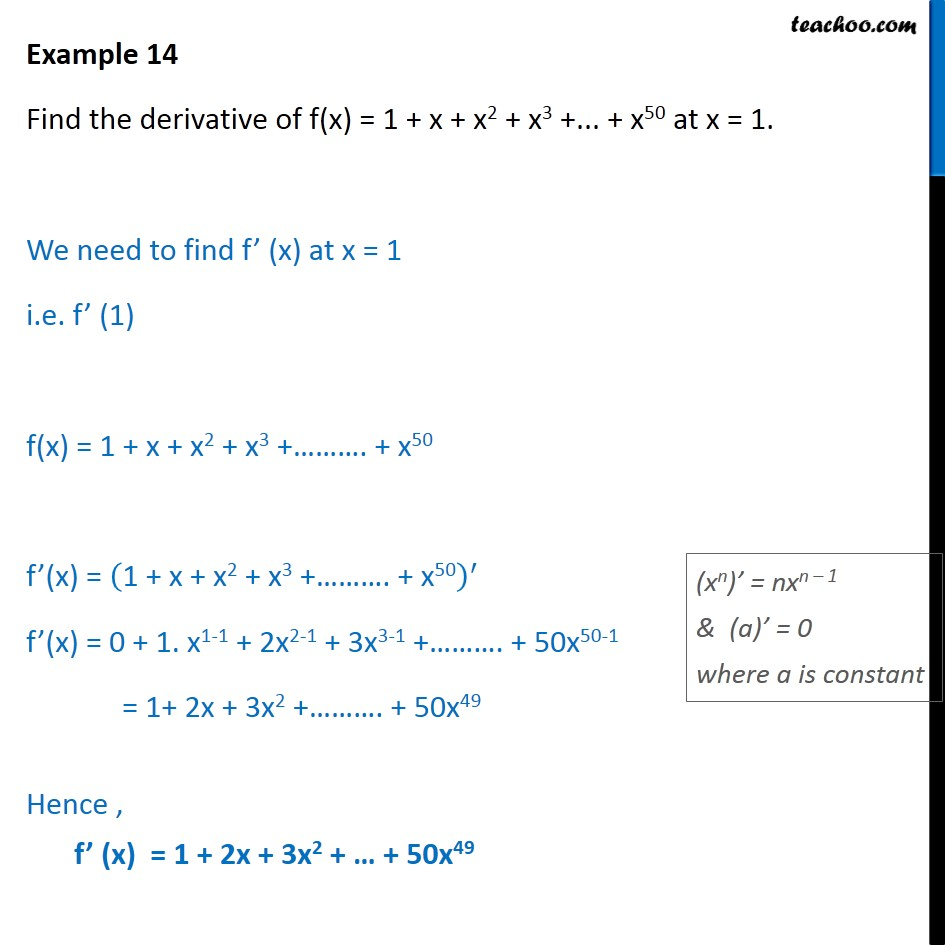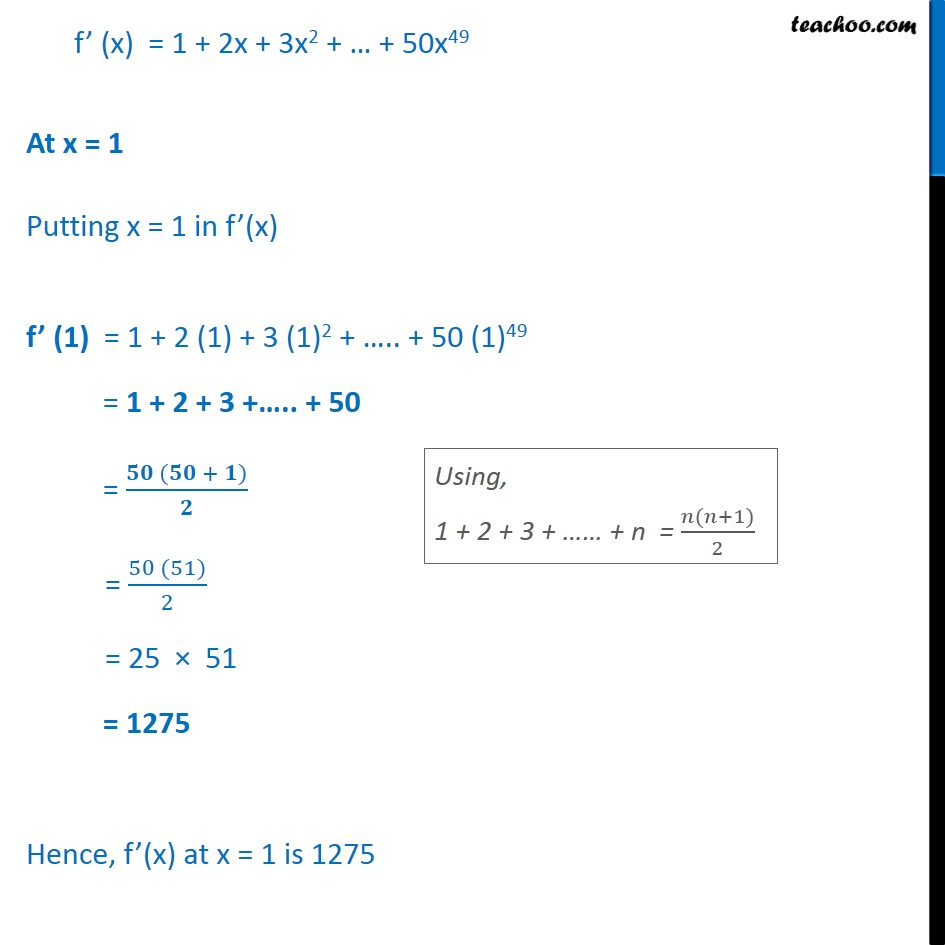1. Chapter 13 Class 11 Limits and Derivatives
2. Serial order wise
3. Examples

Transcript

Example 14 Find the derivative of f(x) = 1 + x + x2 + x3 +... + x50 at x = 1. We need to find f’ (x) at x = 1 i.e. f’ (1) f(x) = 1 + x + x2 + x3 +………. + x50 f’(x) = 1 + x + x2 + x3 +………. + x50﷯﷮′﷯ f’(x) = 0 + 1. x1-1 + 2x2-1 + 3x3-1 +………. + 50x50-1 = 1+ 2x + 3x2 +………. + 50x49 Hence , f’ (x) = 1 + 2x + 3x2 + … + 50x49 f’ (x) = 1 + 2x + 3x2 + … + 50x49 At x = 1 Putting x = 1 in f’(x) f’ (1) = 1 + 2 (1) + 3 (1)2 + ….. + 50 (1)49 = 1 + 2 + 3 +….. + 50 = 𝟓𝟎 (𝟓𝟎 + 𝟏)﷮𝟐﷯ = 50 (51)﷮2﷯ = 25 × 51 = 1275 Hence, f’(x) at x = 1 is 1275

Examples